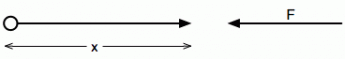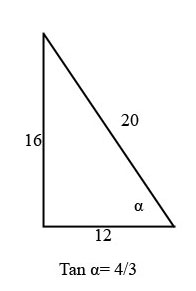I have forgotten

•http://facebook.com/
•https://www.google.com/accounts/o8/id
•https://me.yahoo.com

# Differential

## Introduction

Differential equations are an powerful mathematical tool that help us understand nature and finance, allowing us to make accurate calculations, including:
• movement of an object
• the collision of two cars
• trajectories of planets

As an example:
Imagine a particle that is projected horizontally (Gravity is neglected):• Velocity is given by
• Acceleration is
• It is assumed that the drag is proportional to

Applying Newton's second Law: "Force = Mass x Acceleration"

Therefore if we are interested in the distance x
or

If we are interested in time then:

## Definitions

Particular solutions

• When
and

• When
and

Differential Equations which involve only one independent variable are called Ordinary. In these equations x is the independent variable and y is the dependent variable. For example :

Equations which involve two or more independent variables and partial differential coefficients with respect to them are called Partial. For example :
• When
The Laplace equation

### Order

Equations that involve a second differential coefficient but none of higher orders is said to be Second Order.

• When
and

• When
and

• When

For example :
First order
Second order
Third order

### Degree

The degree of an equation is the power of the highest differential coefficient once the equation has been made rational and integral as far as the differential coefficients are concerned. For example :

• When
and

• When

First degree
Second degree

Note that this definition of degree does not require x or y to occur rationally or integrally.

## The Geometrical Meaning Of A Differential Equation

This section presents geometric characteristics of the solution of a differential equation .

### Linear Solution

A linear function is a function such as where

A quadric function is a function such as where

If then

Therefore
• If A = 0 the graph is as above.
• If A = 1
• If A = -2

### Exponential Solution

An exponential function is a function such as where
Now consider the following equation:

This can be rearranged as:

The variables have now been separated and :

From which the explicit form is given by:

## The Formation Of Differential Equations By Elimination

If from the following equation we eliminate the arbitrary constant we get the following:

Extending this concept, if we started with n arbitrary constants, we could eliminate them by n differentiations. The result would be a differential equation of the order.

Conversley if we are given a differential equation of the order we can, in general, obtain an equivalent relationship containing no derivatives but n arbitrary constants. This relationship is called "The General Solution"

For Example
where w i aconstant

Integrating with respect to x gives

And so on until

Where A, B, C and E are all arbitrary constants

## The Complete Primitive; Particular Integral; And Singular Solution

The solution of a differential equation containing the full number of arbitrary constants is called "The Complete Primative". Any solution derived from the complete Primitive by giving particular values to these constants is called a "A Particular Integral"

For example
A Particular solution of
is given by
(Obtained by putting A,B,C,E = 0) or

Example:
##### Example - The use of Differential Equations to Solve Problems in Dynamics
Problem
A cricket ball is thrown vertically upwards with a velocity of v ft/sec. The retardation is or . Find the maximum height reached (Y) and the time of flight to the vertex (T).

Prove that the Initial velocity u is given by:
Workings
The acceleration = -kv - g

To find the time of Flight T
thus

When t = 0 v = u
Thus

At the vertex t = T and v = 0 so
i.e.
{T = \frac{1}{k}\;Ln\left(\frac{ku}{g} + 1 \right)

For Height Y

But when y = 0, v = u so

At the Vertex v = 0
Solution
The flight time is:
{T = \frac{1}{k}\;Ln\left(\frac{ku}{g} + 1 \right)

Max Height is:

## Differential Equations Which Include Trigonometrical Functions The Right Hand Side

In the following worked examples is usually re-written as . For those unused to this type of trigonometrical manipulation, the following notes should help.
Example:
##### Example - Basic examples .
Problem
Basic trigonometrical examples
Workings
The reference page on Trigonometrical Formulae includes:-

Considering the first equation , this can be re-written as:-

Now if during the solution of a differential equation we arrive at :-

we can compare the right hand side with the right hand side of (5) and we can see that they are of the same form but has been replaced by "3" and by "4". Clearly this can not be correct as the Sine and Cosine can not have a value above unity but if we draw the following right angled triangle.Values of Sine and Cosine can be obtained which can be put into equation (5)

This can be re-arranged to satisfy the requirements of equation (6)

## Mixed Examples

The next examples will present some mixed differential equations (containing exponential, polynomials, sine, cosine).
Example:
##### Example - Using the lambda example
Problem
Solve

Workings

Using the D operator

Solution
Therefore the General Solution is given by:-

## Section Pages

#### Taylor

Computes the first and second derivatives of a function using the Taylor formula.
 double taylor1 (double (*f)(double), double x, double h, double gamma = 1.0)[inline] double taylor2 (double (*f)(double), double x, double h, double gamma = 1.0)[inline]

#### Taylor Table

Computes the first and second derivatives of a function at multiple points.
 std::vector taylor1_table (double (*f)(double), std::vector &points, double h, double gamma = 1.0) std::vector taylor2_table (double (*f)(double), std::vector &points, double h, double gamma = 1.0)

#### First Order

First Order Differential Equations with worked examples

#### Linear with Constant Coefficient

A guide to linear equations of second and higher degrees

#### Separable

This section contains worked examples of the type of differential equation which can be solved by integration

#### The D operator

Solving Differential Equations using the D operator

#### Homogeneous

The solution of homogeneous differential equations including the use of the D operator

#### Linear Simultaneous Equations

Linear simultaneous differential equations

#### Partial

An Introduction to Partial Differential Equations